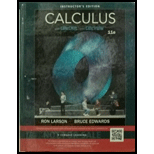Chapter 9.8, Problem 27E### Calculus (MindTap Course List)

11th Edition
Ron Larson + 1 other
ISBN: 9781337275347

#### Solutions

Chapter
Section### Calculus (MindTap Course List)

11th Edition
Ron Larson + 1 other
ISBN: 9781337275347
Textbook Problem

# Finding the Interval of Convergence In Exercise 15-38. find the Interval of convergence of the power series. (Be sure in include a check for convergence at the endpoints of the interval.) ∑ n = 0 ∞ ( − 1 ) n + 1 ( x − 1 ) n + 1 n + 1

To determine

To Calculate: The Interval of convergence for the power series n=0(1)n+1(x1)n+1n+1.

Explanation

Given:

The power series is n=0(1)n+1(x1)n+1n+1.

Formula Used:

By ratio test, the series is convergent if

limn|un+1un|<1

Calculation:

Consider the power series,

n=0(1)n+1(x1)n+1n+1

The nth term of the series is

un=(1)n+1(x1)n+1n+1

The (n+1)th term of the series is

un+1=(1)n+2(x1)n+2n+2

### Still sussing out bartleby?

Check out a sample textbook solution.

See a sample solution

#### The Solution to Your Study Problems

Bartleby provides explanations to thousands of textbook problems written by our experts, many with advanced degrees!

Get Started

## Additional Math Solutions

#### Expand each expression in Exercises 122. (x+1x)2

Finite Mathematics and Applied Calculus (MindTap Course List)

#### In problems 45-62, perform the indicated operations and simplify. 49.

Mathematical Applications for the Management, Life, and Social Sciences

#### Given region RST, explain why ARSTAR+AS+AT.

Elementary Geometry For College Students, 7e